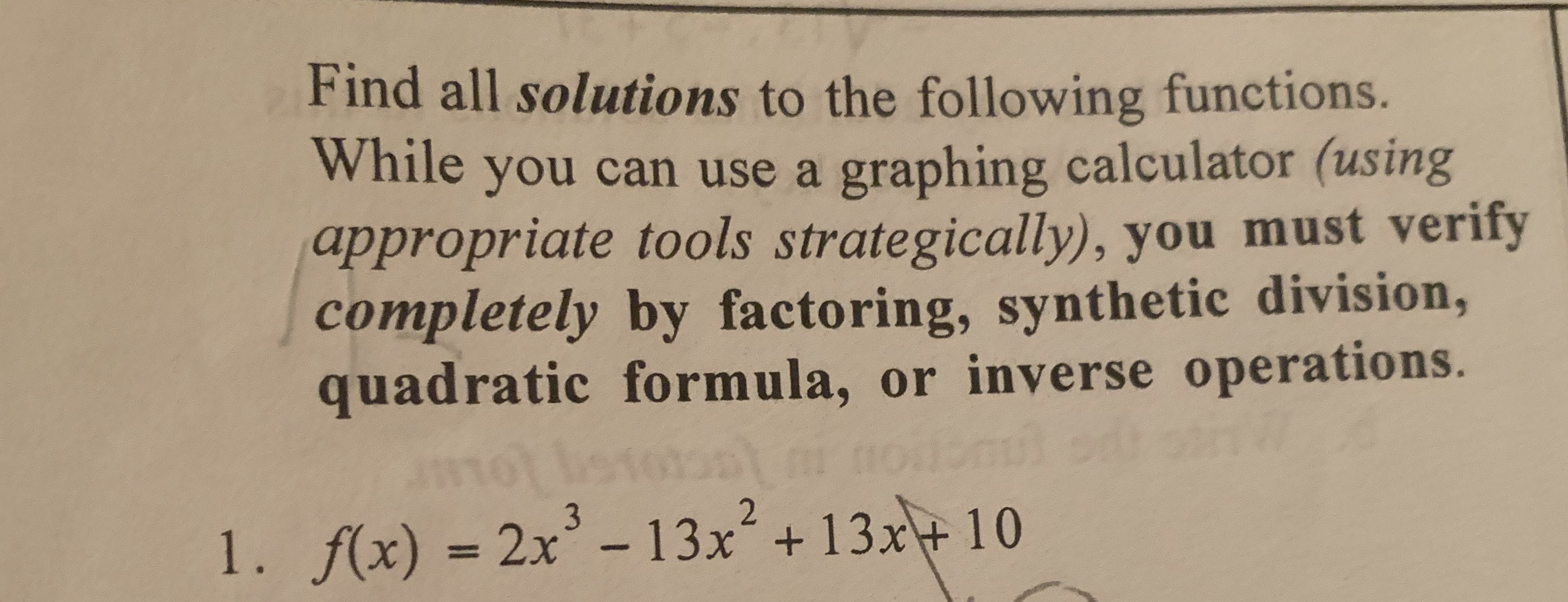# Find all solutions to the following functions. While you can use a graphing calculator (using appropriate tools strategically), you must verify completely by factoring, synthetic division, quadratic formula, or inverse operations. l . /(x) = 2x3-13x2 + 13x + 10

Questionhelp_outlineImage TranscriptioncloseFind all solutions to the following functions. While you can use a graphing calculator (using appropriate tools strategically), you must verify completely by factoring, synthetic division, quadratic formula, or inverse operations. l . /(x) = 2x3-13x2 + 13x + 10 fullscreen

### Want to see the step-by-step answer?